GFG App
Open AppBrowser
Continue

# How to Sort a Pandas DataFrame based on column names or row index?

Pandas dataframe.sort_index() method sorts objects by labels along the given axis. Basically, the sorting algorithm is applied to the axis labels rather than the actual data in the Dataframe and based on that the data is rearranged.

## Creating Pandas Dataframe

Create a DataFrame object from the Python list of tuples with columns and indices, say column names are: ‘Name’, ‘Age’, ‘Place’, and ‘College’.

## Python3

 `# import pandas library as pd` `import` `pandas as pd`   `# List of Tuples` `students ``=` `[(``'Ankit'``, ``22``, ``'Up'``, ``'Geu'``),` `           ``(``'Ankita'``, ``31``, ``'Delhi'``, ``'Gehu'``),` `           ``(``'Rahul'``, ``16``, ``'Tokyo'``, ``'Abes'``),` `           ``(``'Simran'``, ``41``, ``'Delhi'``, ``'Gehu'``),` `           ``(``'Shaurya'``, ``33``, ``'Delhi'``, ``'Geu'``),` `           ``(``'Harshita'``, ``35``, ``'Mumbai'``, ``'Bhu'` `),` `           ``(``'Swapnil'``, ``35``, ``'Mp'``, ``'Geu'``),` `           ``(``'Priya'``, ``35``, ``'Uk'``, ``'Geu'``),` `           ``(``'Jeet'``, ``35``, ``'Guj'``, ``'Gehu'``),` `           ``(``'Ananya'``, ``35``, ``'Up'``, ``'Bhu'``)` `            ``]`   `# Create a DataFrame object from` `# list of tuples with columns` `# and indices.` `details ``=` `pd.DataFrame(students, columns ``=``[``'Name'``, ``'Age'``,` `                                           ``'Place'``, ``'College'``],` `                        ``index ``=``[ ``'b'``, ``'c'``, ``'a'``, ``'e'``, ``'f'``, ``'g'``,` `                                 ``'i'``, ``'j'``, ``'k'``, ``'d'``])` `# show the dataframe` `details`

Output: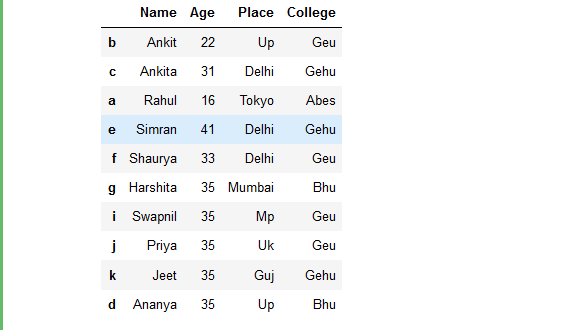## Sort a DataFrame based on row index

Example 1: Sort the Rows of Dataframe based on row index label names, i.e. row index: a, b, c, d, etc.

## Python3

 `# import pandas library as pd` `import` `pandas as pd`   `# sort the rows of dataframe ` `# based on row index` `rslt_df ``=` `details.sort_index()`   `# show the resultant Dataframe` `rslt_df`

Output: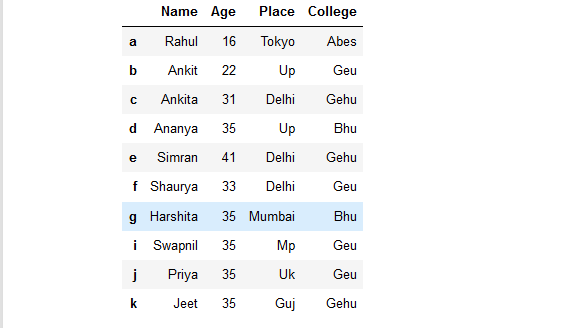Example 2: Sort rows of a Dataframe in Descending Order based on Row index labels.

## Python3

 `# import pandas library as pd` `import` `pandas as pd`   `# sort the rows of dataframe in descending ` `# order based on row index ` `rslt_df ``=` `details.sort_index(ascending ``=` `False``)`   `# show the resultant Dataframe` `rslt_df`

Output: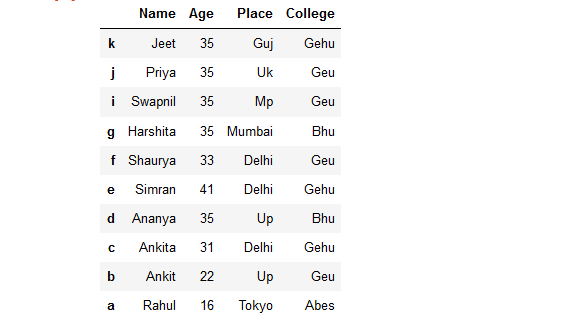Example 3: Sort rows of a Dataframe based on Row index labels inplace=True. It will apply the filter in the Original Dataframne.

## Python3

 `# import pandas library as pd` `import` `pandas as pd`   `# sort the rows of dataframe in Place ` `# based on row index ` `details.sort_index(inplace ``=` `True``)`   `# show the resultant Dataframe` `details`

Output:## Sort a DataFrame based on column names

Example 1: Sort Columns name of a Dataframe based on Column Names, i.e. age, college, name, place.

## Python3

 `# import pandas library as pd` `import` `pandas as pd`   `# sort a dataframe based on column names` `rslt_df ``=` `details.sort_index(axis ``=` `1``)`   `# show the resultant Dataframe` `rslt_df`

Output: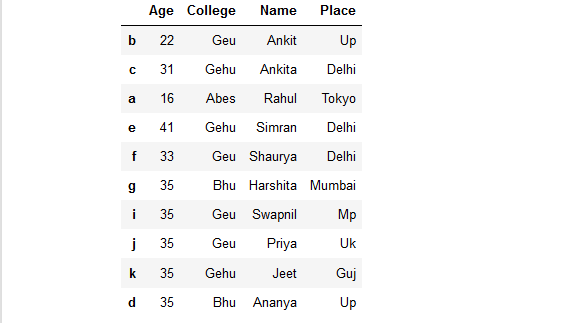Example 2: Sort a Dataframe in descending order based on column names.

## Python3

 `# import pandas library as pd` `import` `pandas as pd`   `# sort a dataframe in descending ` `# order based on column names` `rslt_df ``=` `details.sort_index(ascending ``=` `False``, axis ``=` `1``)`   `# show the resultant Dataframe` `rslt_df`

Output:Example 3: Sort a Dataframe inplace based on column names.

## Python3

 `# import pandas library as pd` `import` `pandas as pd`   `# sort a dataframe in place ` `# based on column names` `details.sort_index(inplace ``=` `True``, axis ``=` `1``)`   `# show the resultant Dataframe` `details`

Output: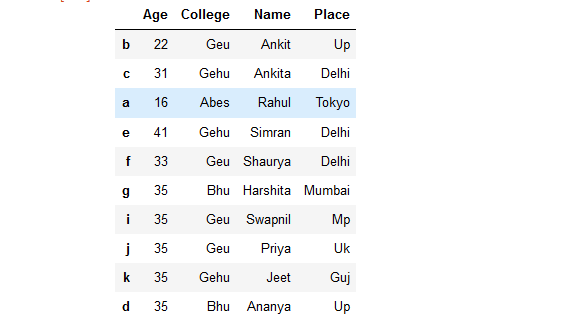My Personal Notes arrow_drop_up